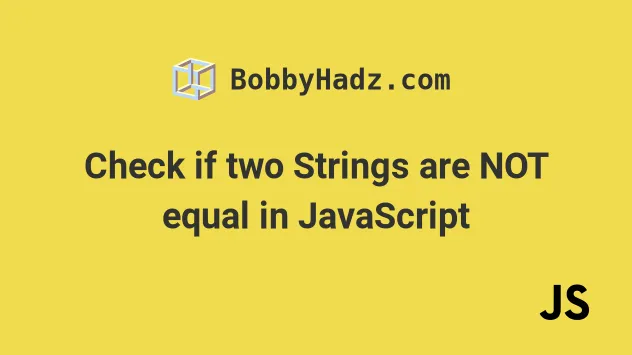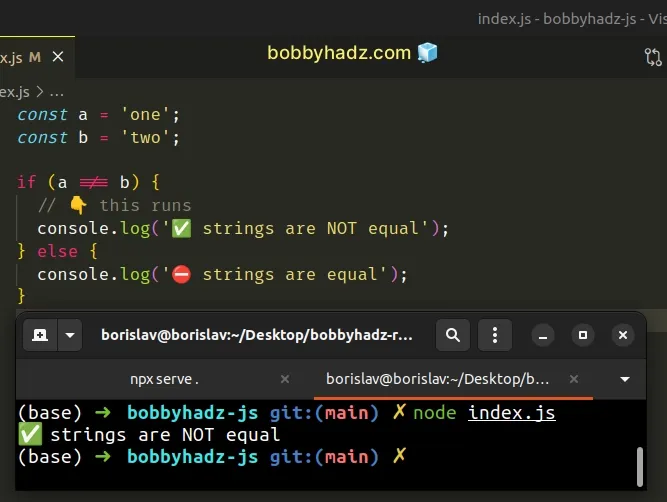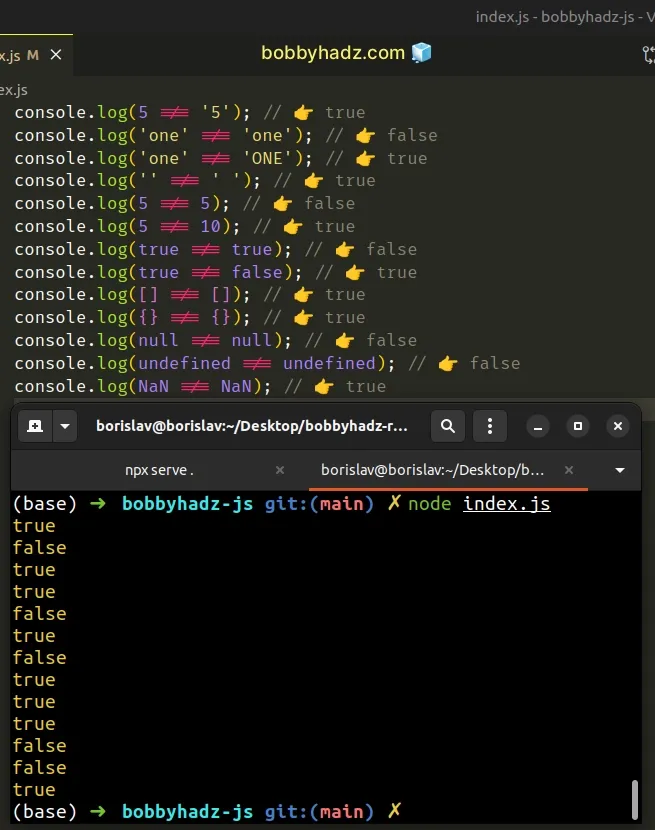# Check if two Strings are NOT equal in JavaScriptLast updated: Dec 27, 2022
2 min## #Check if two Strings are NOT equal

Use the strict inequality (!==) operator to check if two strings are not equal, e.g. `a !== b`.

The strict inequality operator returns `true` if the strings are not equal and `false` otherwise.

index.js
```Copied!```const a = 'one';
const b = 'two';

if (a !== b) {
// 👇️ this runs
console.log('✅ strings are NOT equal');
} else {
console.log('⛔️ strings are equal');
}
``````We used the strict inequality (!==) operator to check if two strings are not equal.

The operator returns a boolean result:

• `true` if the values are not equal
• `false` if the values are equal

The strict inequality (!==) operator is the negation of the strict equality (===) operator.

Here are some more examples of using the strict inequality (!==) operator.

index.js
```Copied!```console.log(5 !== '5'); // 👉️ true
console.log('one' !== 'one'); // 👉️ false
console.log('one' !== 'ONE'); // 👉️ true
console.log('' !== ' '); // 👉️ true
console.log(5 !== 5); // 👉️ false
console.log(5 !== 10); // 👉️ true
console.log(true !== true); // 👉️ false
console.log(true !== false); // 👉️ true
console.log([] !== []); // 👉️ true
console.log({} !== {}); // 👉️ true
console.log(null !== null); // 👉️ false
console.log(undefined !== undefined); // 👉️ false
console.log(NaN !== NaN); // 👉️ true
``````The strict inequality (!==) operator considers two values of different types to be different, as opposed to the loose inequality (!=) operator.

Two values of different types will never be equal when using the strict inequality operator.

It's always recommended to use the strict operators (!==, ===) because they don't try to coerce the values to the same type before comparing them.

The strict operators (!==, ===) produce more predictable results.

Notice that in the last example, `NaN !== NaN` returns `true`. This is because `NaN` (not a number) is the only value in JavaScript that is not equal to itself.

One common use case for the loose equality (==) and loose inequality (!=) operators is when checking if a value is nullish.

index.js
```Copied!```const a = null;

if (a == null) {
// 👇️ this runs
console.log('a is null or undefined');
} else {
console.log('a is not null and undefined');
}

if (a != null) {
console.log('a is not null and undefined');
}
``````

The loose equality operator (==) considers `null` and `undefined` to be equal which is not the case when using the strict equality operator (===).

index.js
```Copied!```console.log(null == undefined); // 👉️ true

console.log(null === undefined); // 👉️ false
``````

This is why the condition `if (a != null)` evaluates to `true` if `a` is not equal to `null` and `undefined`.

This is the most common use case for the loose equality (==, !==) operators in JavaScript.

If you use the loose equality operator to check if two values are not equal, you might get confusing results.

If you want to read more about how the loose equality operators work, check out this section of the MDN docs.

I wrote a book in which I share everything I know about how to become a better, more efficient programmer.You can use the search field on my Home Page to filter through all of my articles.